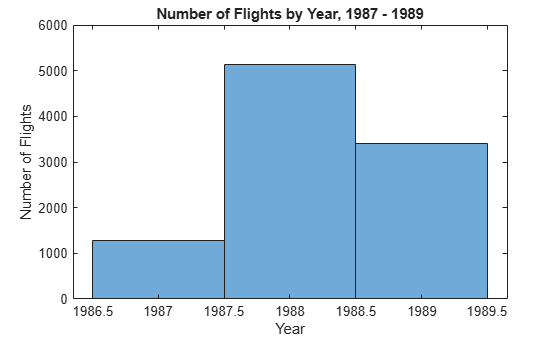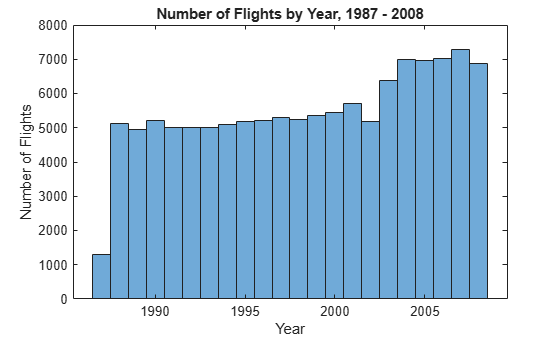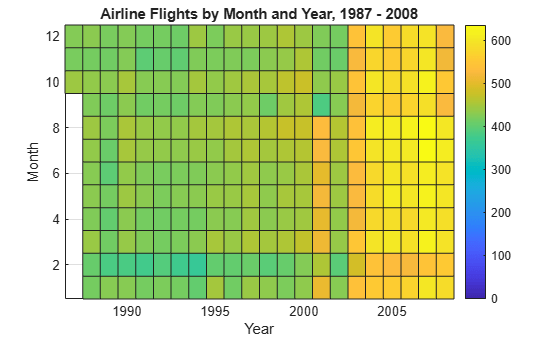# Analyze Big Data in MATLAB Using Tall Arrays

This example shows how to use tall arrays to work with big data in MATLAB®. You can use tall arrays to perform a variety of calculations on different types of data that does not fit in memory. These include basic calculations, as well as machine learning algorithms within Statistics and Machine Learning Toolbox™.

This example operates on a small subset of data on a single computer, and then it then scales up to analyze all of the data set. However, this analysis technique can scale up even further to work on data sets that are so large they cannot be read into memory, or to work on systems like Apache Spark™.

### Introduction to Tall Arrays

Tall arrays and tall tables are used to work with out-of-memory data that has any number of rows. Instead of writing specialized code that takes into account the huge size of the data, tall arrays and tables let you work with large data sets in a manner similar to in-memory MATLAB® arrays. The difference is that `tall` arrays typically remain unevaluated until you request that the calculations be performed.

This deferred evaluation enables MATLAB to combine the queued calculations where possible and take the minimum number of passes through the data. Since the number of passes through the data greatly affects execution time, it is recommended that you request output only when necessary.

### Create datastore for Collection of Files

Creating a datastore enables you to access a collection of data. A datastore can process arbitrarily large amounts of data, and the data can even be spread across multiple files in multiple folders. You can create a datastore for most types of files, including a collection of tabular text files (demonstrated here), spreadsheets, images, a SQL database (Database Toolbox™ required), Hadoop® sequence files, and more.

Create a datastore for a `.csv` file containing airline data. Treat `'NA'` values as missing so that `tabularTextDatastore` replaces them with `NaN` values. Select the variables of interest, and specify a categorical data type for the `Origin` and `Dest` variables. Preview the contents.

```ds = tabularTextDatastore('airlinesmall.csv'); ds.TreatAsMissing = 'NA'; ds.SelectedVariableNames = {'Year','Month','ArrDelay','DepDelay','Origin','Dest'}; ds.SelectedFormats(5:6) = {'%C','%C'}; pre = preview(ds)```
```pre=8×6 table Year Month ArrDelay DepDelay Origin Dest ____ _____ ________ ________ ______ ____ 1987 10 8 12 LAX SJC 1987 10 8 1 SJC BUR 1987 10 21 20 SAN SMF 1987 10 13 12 BUR SJC 1987 10 4 -1 SMF LAX 1987 10 59 63 LAX SJC 1987 10 3 -2 SAN SFO 1987 10 11 -1 SEA LAX ```

### Create Tall Array

Tall arrays are similar to in-memory MATLAB arrays, except that they can have any number of rows. Tall arrays can contain data that is numeric, logical, datetime, duration, calendarDuration, categorical, or strings. Also, you can convert any in-memory array to a tall array. (The in-memory array `A` must be one of the supported data types.)

The underlying class of a tall array is based on the type of datastore that backs it. For example, if the datastore `ds` contains tabular data, then `tall(ds)` returns a tall table containing the data.

`tt = tall(ds)`
```tt = Mx6 tall table Year Month ArrDelay DepDelay Origin Dest ____ _____ ________ ________ ______ ____ ? ? ? ? ? ? ? ? ? ? ? ? ? ? ? ? ? ? : : : : : : : : : : : : ```

The display indicates the underlying data type and includes the first several rows of data. The size of the table displays as "Mx6" to indicate that MATLAB does not yet know how many rows of data there are.

### Perform Calculations on Tall Arrays

You can work with tall arrays and tall tables in a similar manner in which you work with in-memory MATLAB arrays and tables.

One important aspect of tall arrays is that as you work with them, MATLAB does not perform most operations immediately. These operations appear to execute quickly, because the actual computation is deferred until you specifically request output. This deferred evaluation is important because even a simple command like `size(X)` executed on a tall array with a billion rows is not a quick calculation.

As you work with tall arrays, MATLAB keeps track of all of the operations to be carried out and optimizes the number of passes through the data. Thus, it is normal to work with unevaluated tall arrays and request output only when you require it. MATLAB does not know the contents or size of unevaluated tall arrays until you request that the array be evaluated and displayed.

Calculate the mean departure delay.

`mDep = mean(tt.DepDelay,'omitnan')`
```mDep = tall double ? ```

### Gather Results into Workspace

The benefit of deferred evaluation is that when the time comes for MATLAB to perform the calculations, it is often possible to combine the operations in such a way that the number of passes through the data is minimized. So, even if you perform many operations, MATLAB only makes extra passes through the data when absolutely necessary.

The `gather` function forces evaluation of all queued operations and brings the resulting output back into memory. Since `gather` returns the entire result in MATLAB, you should make sure that the result will fit in memory. For example, use `gather` on tall arrays that are the result of a function that reduces the size of the tall array, such as `sum`, `min`, `mean`, and so on.

Use `gather` to calculate the mean departure delay and bring the answer into memory. This calculation requires a single pass through the data, but other calculations might require several passes through the data. MATLAB determines the optimal number of passes for the calculation and displays this information at the command line.

`mDep = gather(mDep)`
```Evaluating tall expression using the Local MATLAB Session: - Pass 1 of 2: Completed in 1.2 sec - Pass 2 of 2: Completed in 0.85 sec Evaluation completed in 2.7 sec ```
```mDep = 8.1860 ```

### Select Subset of Tall Array

You can extract values from a tall array by subscripting or indexing. You can index the array starting from the top or bottom, or by using a logical index. The functions `head` and `tail` are useful alternatives to indexing, enabling you to explore the first and last portions of a tall array. Gather both variables at the same time to avoid extra passes through the data.

```h = head(tt); tl = tail(tt); [h,tl] = gather(h,tl)```
```Evaluating tall expression using the Local MATLAB Session: - Pass 1 of 1: Completed in 0.77 sec Evaluation completed in 0.95 sec ```
```h=8×6 table Year Month ArrDelay DepDelay Origin Dest ____ _____ ________ ________ ______ ____ 1987 10 8 12 LAX SJC 1987 10 8 1 SJC BUR 1987 10 21 20 SAN SMF 1987 10 13 12 BUR SJC 1987 10 4 -1 SMF LAX 1987 10 59 63 LAX SJC 1987 10 3 -2 SAN SFO 1987 10 11 -1 SEA LAX ```
```tl=8×6 table Year Month ArrDelay DepDelay Origin Dest ____ _____ ________ ________ ______ ____ 2008 12 14 1 DAB ATL 2008 12 -8 -1 ATL TPA 2008 12 1 9 ATL CLT 2008 12 -8 -4 ATL CLT 2008 12 15 -2 BOS LGA 2008 12 -15 -1 SFO ATL 2008 12 -12 1 DAB ATL 2008 12 -1 11 ATL IAD ```

Use `head` to select a subset of 10,000 rows from the data for prototyping code before scaling to the full data set.

`ttSubset = head(tt,10000);`

### Select Data by Condition

You can use typical logical operations on tall arrays, which are useful for selecting relevant data or removing outliers with logical indexing. The logical expression creates a tall logical vector, which then is used to subscript, identifying the rows where the condition is true.

Select only the flights out of Boston by comparing the elements of the categorical variable `Origin` to the value `'BOS'`.

```idx = (ttSubset.Origin == 'BOS'); bosflights = ttSubset(idx,:)```
```bosflights = 207x6 tall table Year Month ArrDelay DepDelay Origin Dest ____ _____ ________ ________ ______ ____ 1987 10 -8 0 BOS LGA 1987 10 -13 -1 BOS LGA 1987 10 12 11 BOS BWI 1987 10 -3 0 BOS EWR 1987 10 -5 0 BOS ORD 1987 10 31 19 BOS PHL 1987 10 -3 0 BOS CLE 1987 11 5 5 BOS STL : : : : : : : : : : : : ```

You can use the same indexing technique to remove rows with missing data or NaN values from the tall array.

```idx = any(ismissing(ttSubset),2); ttSubset(idx,:) = [];```

### Determine Largest Delays

Due to the nature of big data, sorting all of the data using traditional methods like `sort` or `sortrows` is inefficient. However, the `topkrows` function for tall arrays returns the top `k` rows in sorted order.

Calculate the top 10 greatest departure delays.

```biggestDelays = topkrows(ttSubset,10,'DepDelay'); biggestDelays = gather(biggestDelays)```
```Evaluating tall expression using the Local MATLAB Session: Evaluation completed in 0.065 sec ```
```biggestDelays=10×6 table Year Month ArrDelay DepDelay Origin Dest ____ _____ ________ ________ ______ ____ 1988 3 772 785 ORD LEX 1989 3 453 447 MDT ORD 1988 12 397 425 SJU BWI 1987 12 339 360 DEN STL 1988 3 261 273 PHL ROC 1988 7 261 268 BWI PBI 1988 2 257 253 ORD BTV 1988 3 236 240 EWR FLL 1989 2 263 227 BNA MOB 1989 6 224 225 DFW JAX ```

### Visualize Data in Tall Arrays

Plotting every point in a big data set is not feasible. For that reason, visualization of tall arrays involves reducing the number of data points using sampling or binning.

Visualize the number of flights per year with a histogram. The visualization functions pass through the data and immediately evaluate the solution when you call them, so `gather` is not required.

`histogram(ttSubset.Year,'BinMethod','integers')`
```Evaluating tall expression using the Local MATLAB Session: Evaluation completed in 0.63 sec ```
```xlabel('Year') ylabel('Number of Flights') title('Number of Flights by Year, 1987 - 1989')```### Scale to Entire Data Set

Instead of using the smaller data returned from `head`, you can scale up to perform the calculations on the entire data set by using the results from `tall(ds)`.

```tt = tall(ds); idx = any(ismissing(tt),2); tt(idx,:) = []; mnDelay = mean(tt.DepDelay,'omitnan'); biggestDelays = topkrows(tt,10,'DepDelay'); [mnDelay,biggestDelays] = gather(mnDelay,biggestDelays)```
```Evaluating tall expression using the Local MATLAB Session: - Pass 1 of 2: Completed in 0.62 sec - Pass 2 of 2: Completed in 1.1 sec Evaluation completed in 2 sec ```
```mnDelay = 8.1310 ```
```biggestDelays=10×6 table Year Month ArrDelay DepDelay Origin Dest ____ _____ ________ ________ ______ ____ 1991 3 -8 1438 MCO BWI 1998 12 -12 1433 CVG ORF 1995 11 1014 1014 HNL LAX 2007 4 914 924 JFK DTW 2001 4 887 884 MCO DTW 2008 7 845 855 CMH ORD 1988 3 772 785 ORD LEX 2008 4 710 713 EWR RDU 1998 10 679 673 MCI DFW 2006 6 603 626 ABQ PHX ```
`histogram(tt.Year,'BinMethod','integers')`
```Evaluating tall expression using the Local MATLAB Session: - Pass 1 of 2: Completed in 1.4 sec - Pass 2 of 2: Completed in 0.81 sec Evaluation completed in 2.5 sec ```
```xlabel('Year') ylabel('Number of Flights') title('Number of Flights by Year, 1987 - 2008')```Use `histogram2` to further break down the number of flights by month for the whole data set. Since the bins for `Month` and `Year` are known ahead of time, specify the bin edges to avoid an extra pass through the data.

```year_edges = 1986.5:2008.5; month_edges = 0.5:12.5; histogram2(tt.Year,tt.Month,year_edges,month_edges,'DisplayStyle','tile')```
```Evaluating tall expression using the Local MATLAB Session: - Pass 1 of 1: Completed in 0.9 sec Evaluation completed in 0.98 sec ```
```colorbar xlabel('Year') ylabel('Month') title('Airline Flights by Month and Year, 1987 - 2008')```### Data Analytics and Machine Learning with Tall Arrays

You can perform more sophisticated statistical analysis on tall arrays, including calculating predictive analytics and performing machine learning, using the functions in Statistics and Machine Learning Toolbox™.

For more information, see Analysis of Big Data with Tall Arrays (Statistics and Machine Learning Toolbox).

### Scale to Big Data Systems

A key capability of tall arrays in MATLAB is the connectivity to big data platforms, such as computing clusters and Apache Spark™.

This example only scratches the surface of what is possible with tall arrays for big data. See Extend Tall Arrays with Other Products for more information about using:

• Statistics and Machine Learning Toolbox™

• Database Toolbox™

• Parallel Computing Toolbox™

• MATLAB® Parallel Server™

• MATLAB Compiler™